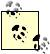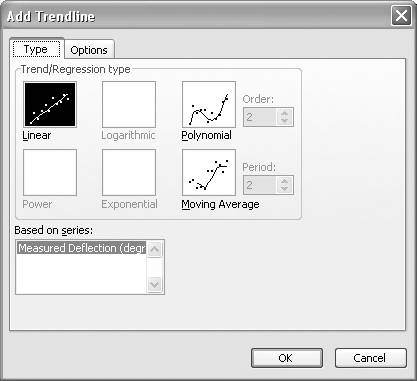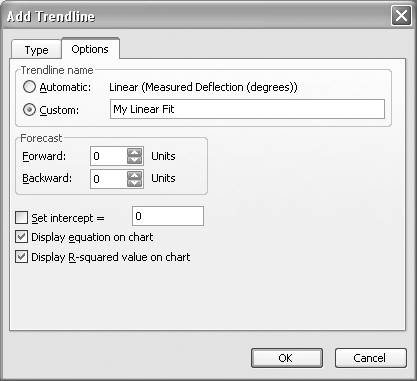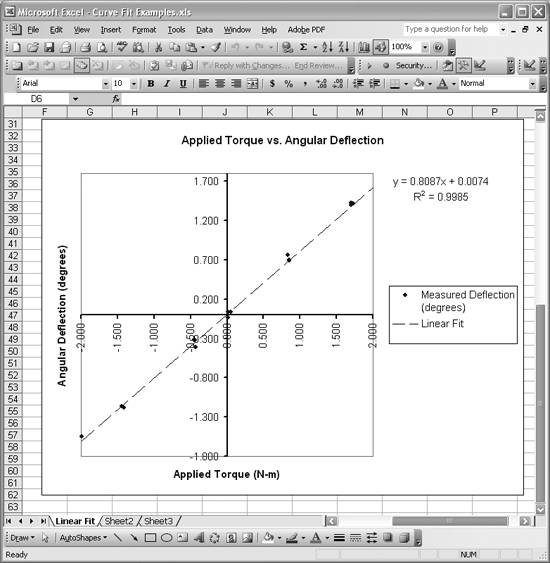# Performing Linear Curve Fitting Using Excel Charts

Problem

You'd like to generate a best-fit straight line for a set of data.

Solution

Use Excel's chart trendline feature to perform a linear curve fit of your data. Plot your data using an XY scatter chart (see Chapter 4). Once your chart has been created, right-click on the data series and select Add Trendline from the pop-up menu.

Discussion

Linear curve fits are easily generated using the trendline feature built into Excel's XY scatter chart. Once you've plotted your data using an XY scatter chart, you can generate a trendline that will be displayed on your chart, superimposed over your data. You can also include the resulting equation for the best-fit line on your chart.

Consider the data shown in Table 8-1. This data represents experimentally obtained angular deflections of an angular spring resulting from prescribed applied torques.

Table 8-1. Angular spring data

Applied torque

Measured deflection

(N-m)

(degrees)

-1.993

-1.547

-1.411

-1.178

-1.440

-1.167

-0.443

-0.318

-0.428

-0.416

-0.444

-0.327

0.016

-0.035

0.013

0.032

0.049

0.036

0.830

0.762

0.854

0.687

0.851

0.698

1.730

1.412

1.703

1.399

1.698

1.419

2.044

1.675

2.063

1.624

2.034

1.627

This data should exhibit a linear trend when plotted. (Assuming the spring obeys Hooke's law.) Indeed this is the case, as is revealed by plotting this data on an XY scatter chart. Once that chart has been created, right-click on the data series and select Add Trendline from the pop-up menu. This will open the Add Trendline dialog box shown in Figure 8-1.Excel's trendline feature allows you to add nonlinear trendlines as well. See Recipe 8.5 for more information.

Select the Linear Trend/Regression type as shown. Before pressing OK, click the Options tab (see Figure 8-2).

Select "Display equation on chart" and "Display R-squared value on chart." The former will display the resulting best-fit equation on your chart, while the latter will also include the R-squared value, allowing you to assess the goodness of the fit (see Recipe 8.7). Press OK to go back to your chart and see the resulting trendline.

Figure 8-1. Add Trendline dialog boxFigure 8-2. Add Trendline Options tabFigure 8-3 shows the resulting best-fit line for the example data contained in Table 8-1. The best-fit line is shown as the dashed line in Figure 8-3. The original data points are shown as dots. Clearly the data follows a straight line trend very well.

Figure 8-3. Linear fitExcel's trendline feature can give you poor results if your data consists of very large numbers that differ only slightly relative to their magnitudes (for example, if your y-values look something like 100,000,001, 100,000,001.5, 100,000,002.3, and so on). If your data looks like this, then you should center it or scale it before performing any sort of curve fit. You can easily center data by subtracting the mean value from each data point.

The equation for the best-fit line is displayed in the upper-right of the chart. The R-squared value for the trendline is very close to 1, indicating a good fit.You can edit your trendline by selecting it and pressing Ctrl-1. To delete the trendline, select it and then right-click. Choose Clear from the pop-up menu to get rid of it.

In practice, you could use the slope from this best-fit line to estimate the spring constant for the spring being tested. You have to be careful with units here, because we've plotted deflection versus torque, resulting in units for the slope of degrees per N-m.

In reality, you'd probably want the reciprocal of this value, to yield a slope with units of N-m per degree, following the standard units for spring constants. You can force your best-fit line to go through the origin by specifying the intercept on the Options tab of the Add Trendline dialog box (see Figure 8-2). Perhaps a better approach would be to plot the angular deflections on the horizontal axis with the torque data on the vertical axis, and then fit a line through the points.The trendline equation shown on the chart in Figure 8-3 uses Y as the dependent variable and X as the independent variable. These correspond to angular deflection and applied torque, respectively, for the example considered here. To make your chart less confusing, you can edit the equation text shown on the chart, changing Y to angular deflection and X to applied torque. Simply select the equation and then click again to position the cursor where you'd like to start editing the text. Then make your changes. Take a look at Figure 8-5 for an example.

See Chapter 4 for recipes covering creating and manipulating charts in Excel. Take a look at Recipe 8.7 to learn more about the R-squared value of trendlines. Also, check out the other recipes in this chapter to learn about alternative methods of performing linear curve fitting.Excel Scientific and Engineering Cookbook (Cookbooks (OReilly))
ISBN: 0596008791
EAN: 2147483647
Year: N/A
Pages: 206
Authors: David M Bourg

Similar book on Amazon